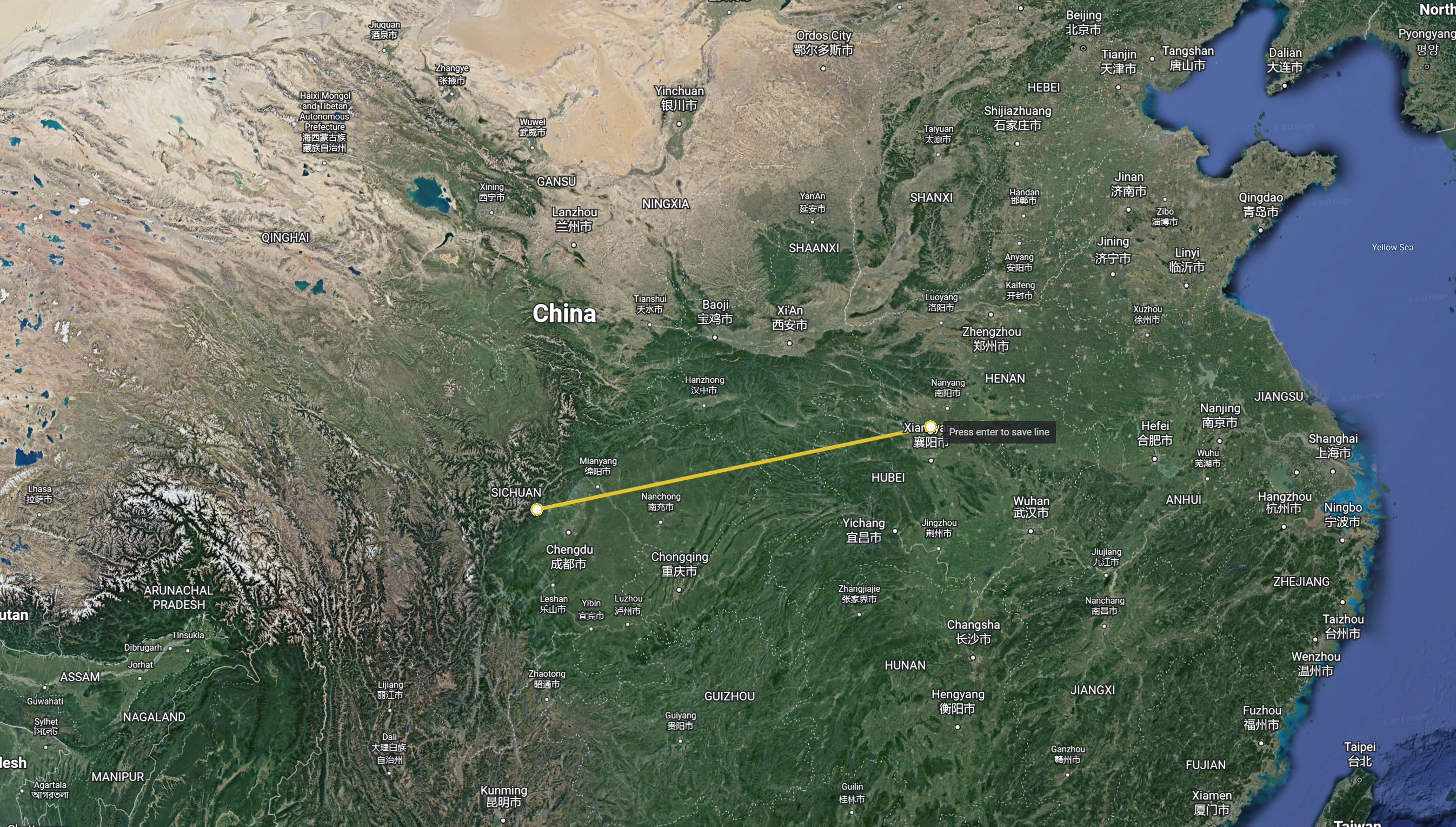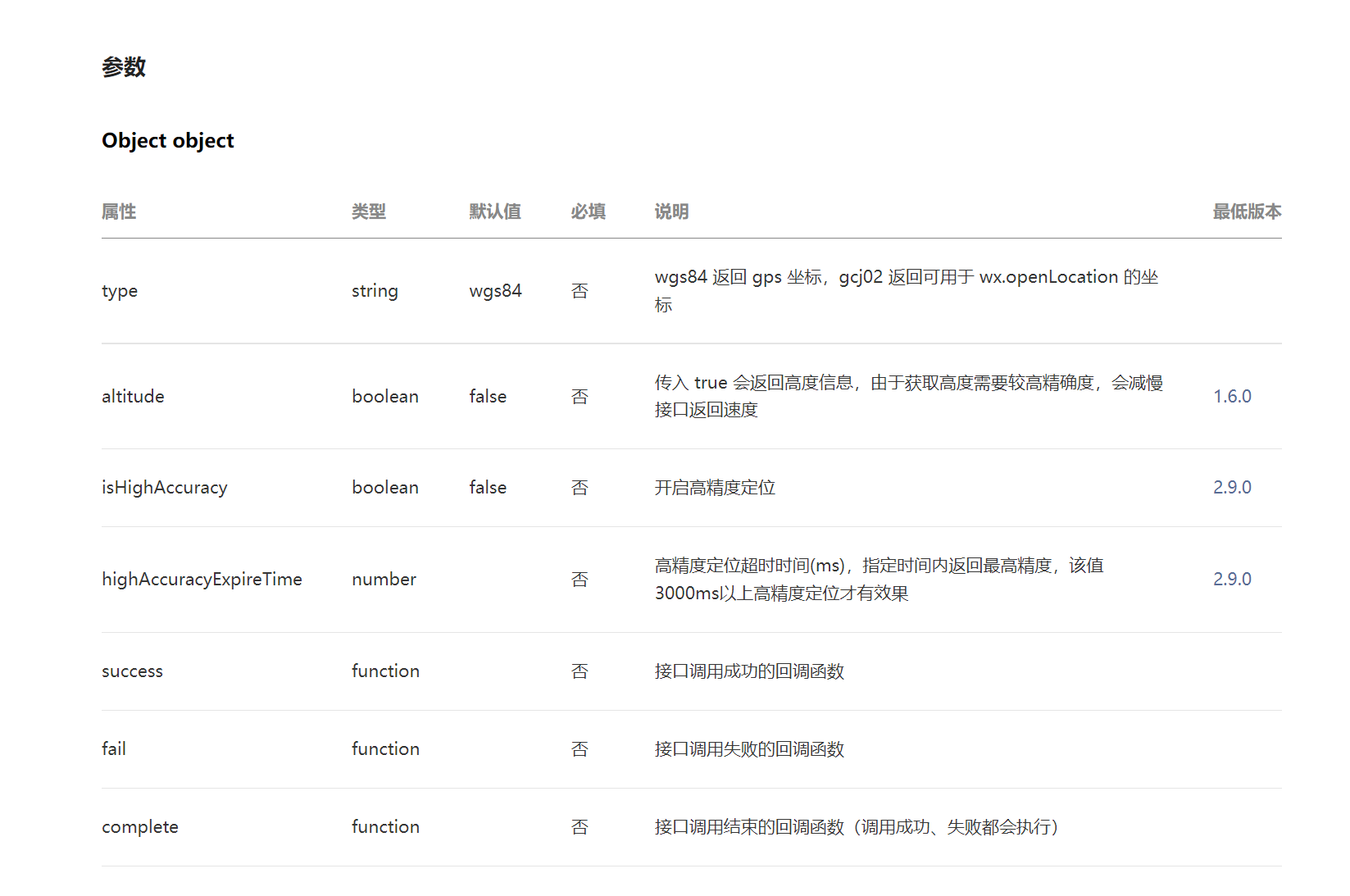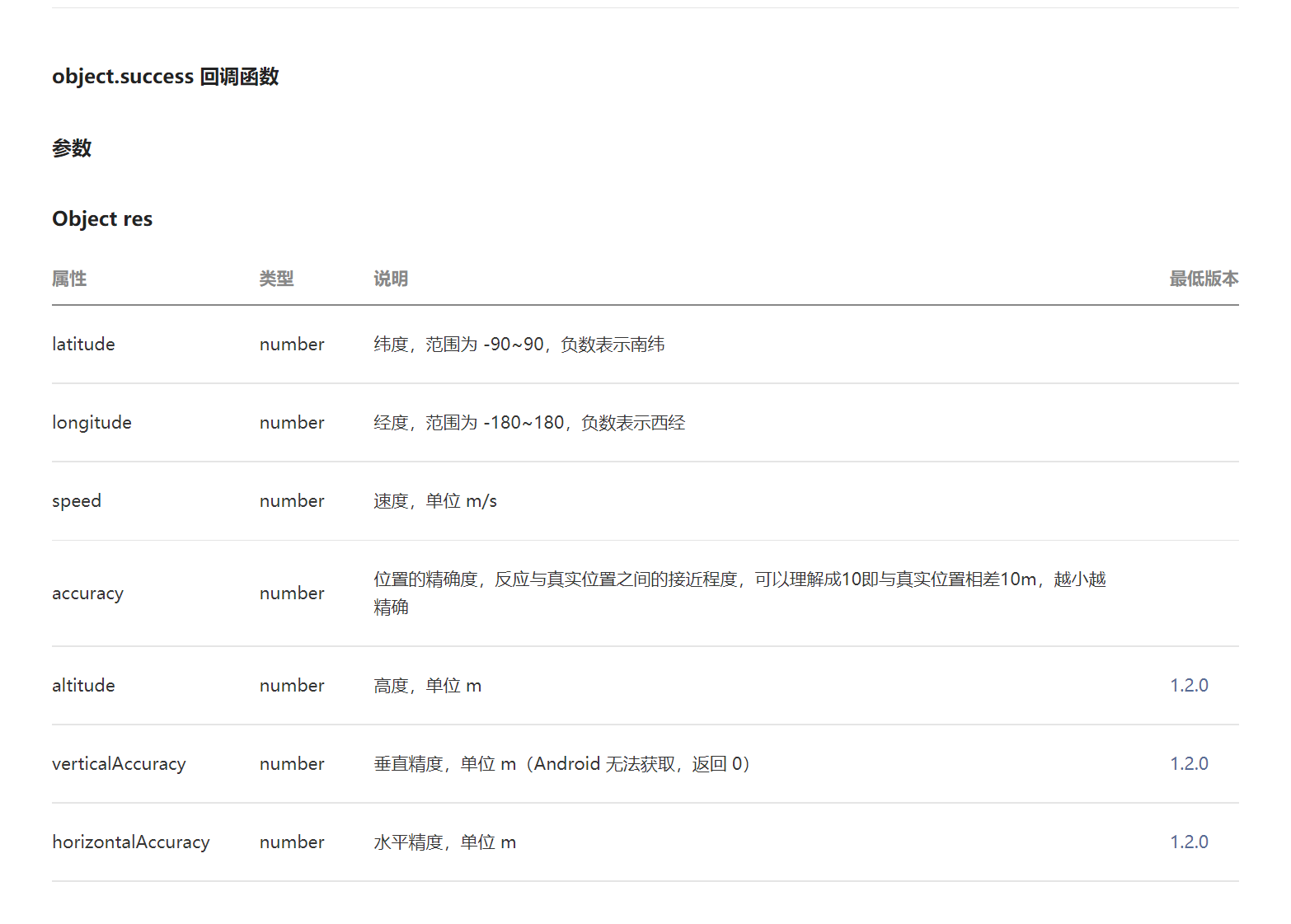# 如何计算地图上两点距离

### 计算地图两点之间距离## 微信小程序启用位置功能需要现在app.json启用地理位置功能

``````"permission": {
"scope.userLocation": {
"desc": "你的位置信息将用于小程序位置接口的效果展示"
}
}
``````

## 获取当前地理位置信息

``````  onShow: function () {
var that = this
wx.getLocation({
type: 'wgs84',
success(res) {
const latitude = res.latitude //维度
const longitude = res.longitude //经度
var lat2 = 34.224167   // 这个是目的地经纬度
var lng2 = 108.941944   // 这个是目的地经纬度
let space = that.space(latitude, longitude, lat2, lng2)
console.log('我跟小寨之间的距离是：', space, '千米')
}
})
``````

## 接口调用入参## 成功回调函数## 计算位置的方法 返回数据为千米

``````  /**

* caculate the great circle distance

* @param {Object} lat1 维度1

* @param {Object} lng1 经度1

* @param {Object} lat2 维度2

* @param {Object} lng2 经度2

*/
space(lat1, lng1, lat2, lng2) {
console.log(lat1, lng1, lat2, lng2)
var radLat1 = lat1 * Math.PI / 180.0;
var radLat2 = lat2 * Math.PI / 180.0;
var b = lng1 * Math.PI / 180.0 - lng2 * Math.PI / 180.0;
var s = 2 * Math.asin(Math.sqrt(Math.pow(Math.sin(a / 2), 2) +
Math.cos(radLat1) * Math.cos(radLat2) * Math.pow(Math.sin(b / 2), 2)));
s = s * 6378.137;
s = Math.round(s * 10000) / 10000;
return s // 单位千米
},
``````

## 如何获取目的地经纬度?

### 通过谷歌地图获取 或者 其他方式 然后把度分秒格式的经纬度转换成常用表示方式https://www.17ditu.com/tool/convert/

目录